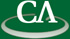## Ben-Or/Tiwari interpolation using discrete logarithms.

### Michael Monagan, Centre for Experimental and Constructive Mathematics, SFU

July 9th at 2:30pm in K9509.

```We present Michael Ben-Or and Prasoon Tiwari's deterministic algorithm
from 1988 for interpolating a sparse multivariate polynomial f
in n variables over a field k of characteristic 0.
If f has t non-zero terms and we know a term bound T>t
then the algorithm interpolates f using 2T points.

The algorithm works over a field of characteristic p if p > pn^d
where pn is the n'th prime and d = deg(f).
We modify the algorithm to work for p > (1+d)^n where p is
chosen to be a smooth prime, i.e., p-1 has no large prime factors.
```